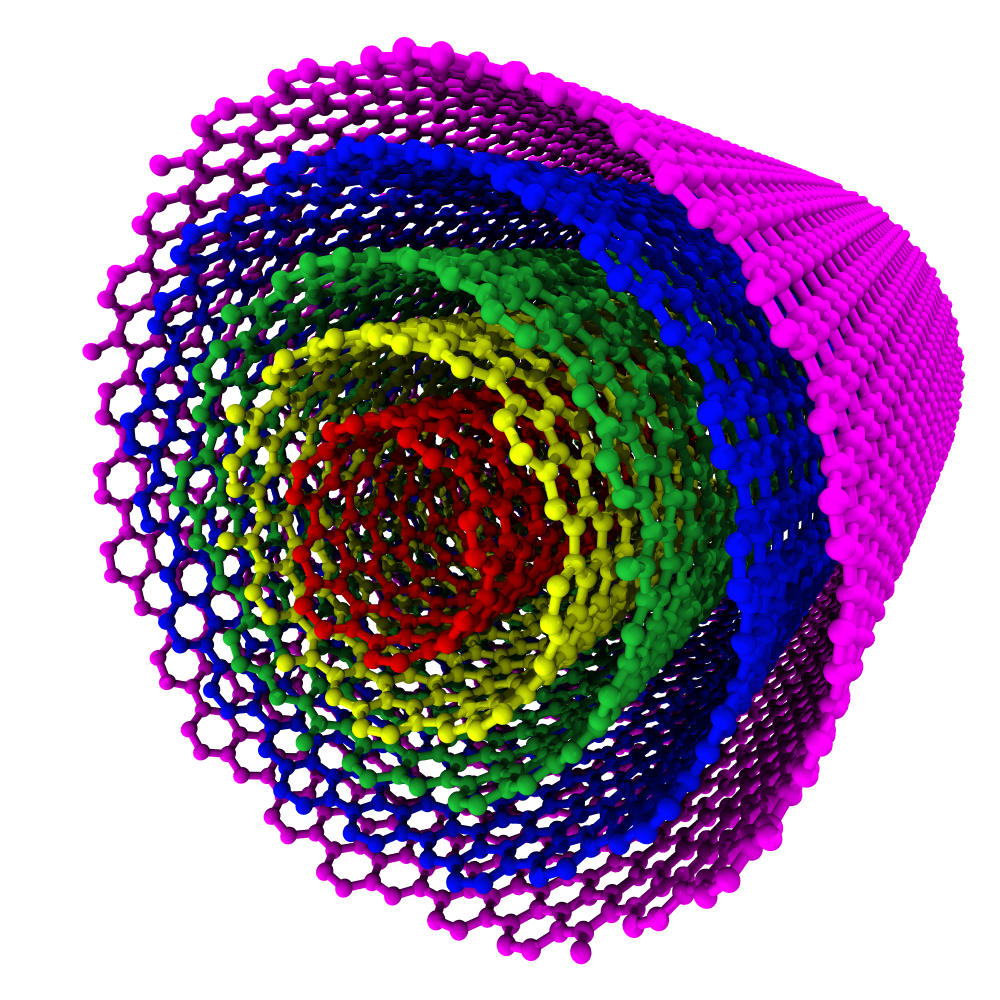# sknano.generators.MWNTGenerator¶

class sknano.generators.MWNTGenerator(*args, *, autogen=True, **kwargs)[source][source]

Class for generating single, MWNT.

Changed in version 0.2.20: MWNTGenerator no longer generates MWNT bundles, only single MWNTs. To generate bundled MWNT structure data, use the MWNTBundleGenerator class.

New in version 0.2.8.

Parameters: Ch_list : list, optional (n, m) for each SWNT wall in MWNT. Nwalls : int, optional Number of SWNT walls in MWNT. Lz : float, optional MWNT length in nanometers. min_wall_diameter : float, optional Minimum MWNT wall diameter, in units of Angstroms. max_wall_diameter : float, optional Maximum MWNT wall diameter, in units of Angstroms. max_walls : int, optional Maximum number of MWNT walls. chiral_types : {None, ‘armchair’, ‘zigzag’, ‘achiral’, ‘chiral’}, optional If None, the chiral_type of each MWNT walls will be random and determined by the set of randomly selected chiral indices (n, m). wall_spacing : float, optional Inter-wall spacing in units of Angstroms. Default value is the van der Waals interaction distance of 3.4 Angstroms. autogen : bool, optional if True, automatically call generate. basis : {list}, optional List of strs of element symbols or atomic number of the two atom basis (default: [‘C’, ‘C’]) New in version 0.3.10. element1, element2 : {str, int}, optional Element symbol or atomic number of basis Atom 1 and 2 Deprecated since version 0.3.10: Use basis instead bond : float, optional $$\mathrm{a}_{\mathrm{CC}} =$$ distance between nearest neighbor atoms, in units of Angstroms. verbose : bool, optional if True, show verbose output

Examples

>>> from sknano.generators import MWNTGenerator
>>> MWNTGenerator(Nwalls=5, min_wall_diameter=10, Lz=5).save()


The above command generated a 5 wall, 10 nanometer long MWNT. The only constraints on the MWNT wall chiralities were the diameter constraints imposed by the min_wall_diameter parameter, which set the minimum wall diameter to 10 Angstroms, as well as the minimum wall-to-wall separation, which defaults to the van der Waals distance of 3.4 Angstroms.

This MWNT chirality may be written as:

$$\mathbf{C}_{\mathrm{h}} = (8,7)@(17,8)@(9,24)@(27,18)@(22,32)$$

Here’s a colorful rendering of the generated MWNT structure:Attributes

 Ch_list Lz_list MWNT length $$L_z = L_{\mathrm{tube}}$$ in nanometers. Natoms Number of atoms in MWNT. Natoms_list List of MWNT SWNT wall’s number of atoms Natoms. Natoms_per_tube Number of atoms in MWNT. Natoms_per_wall Alias for MWNT.Natoms_list Ntubes Number of MWNTs. Nwalls Number of MWNT walls. T_list Length of MWNT unit cell $$|\mathbf{T}|$$ in Å. atoms Structure StructureAtoms. basis NanoStructureBase basis atoms. chiral_set Set of all chiral types in MWNT. chiral_types List of chiral types for each MWNT wall. crystal_cell Structure CrystalCell. dt MWNT wall diameters $$d_t=\frac{|\mathbf{C}_h|}{\pi}$$ in Å. dt_list List of MWNT SWNT wall diameters dt $$d_t=\frac{|\mathbf{C}_h|}{\pi}$$ in Å. element1 Basis element 1 element2 Basis element 2 fmtstr Format string. lattice Structure Crystal3DLattice. max_wall_diameter max_walls min_wall_diameter nz_list Number of nanotube unit cells along the $$z$$-axis. rt MWNT wall radii $$r_t=\frac{|\mathbf{C}_h|}{2\pi}$$ in Å. rt_list List of MWNT SWNT wall radii rt $$r_t=\frac{|\mathbf{C}_h|}{2\pi}$$ in Å. scaling_matrix CrystalCell.scaling_matrix. structure Pointer to self. structure_data Alias for BaseStructureMixin.structure. tube_mass MWNT mass in grams. unit_cell Structure UnitCell. vdw_distance van der Waals distance. vdw_radius van der Waals radius wall_diameters Alias for MWNTMixin.dt_list. wall_radii Alias for MWNTMixin.rt_list. wall_spacing walls List of MWNT SWNT wall structures.

Methods

 clear() Clear list of BaseStructureMixin.atoms. generate() Generate structure data. generate_Ch_list([Nwalls, max_walls, ...]) generate_dt_mask(dt[, max_dt_diff]) Generate boolean mask array. generate_fname([Ch_list, Nwalls]) get_wall(Ch) Return the SWNT structure with chirality Ch. make_supercell(scaling_matrix[, wrap_coords]) Make supercell. read_data(*args, **kwargs) read_dump(*args, **kwargs) read_xyz(*args, **kwargs) rotate(**kwargs) Rotate crystal cell lattice, basis, and unit cell. save([fname, outpath, structure_format, ...]) Save structure data. todict() Return dict of MWNT attributes. transform_lattice(scaling_matrix[, ...]) translate(t[, fix_anchor_points]) Translate crystal cell basis. update_Ch_list([Nwalls, min_wall_diameter, ...]) write_data(**kwargs) write_dump(**kwargs) write_xyz(**kwargs)